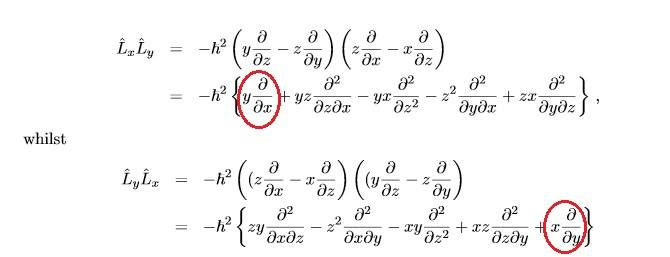# Angular momentum commutation relation, extra terms?

• rwooduk

## Homework Statement

What is the commutation relation between the x and y components of angular momentum L = r X P

None.

## The Attempt at a Solution

I do r X p and get the angular momentum componants:

$$L_{x} = (-i \hbar) (y \frac{d}{dz} - z \frac{d}{dy})$$
$$L_{y} = (-i \hbar) (z \frac{d}{dx} - x \frac{d}{dz})$$
$$L_{z} = (-i \hbar) (x \frac{d}{dy} - y \frac{d}{dx})$$

then when I attempt to put into the commutation relation [Lx,Ly] it comes out very complicated.

My question:

I found a derivation BUT it has extra terms in it (circled in red)Why are there 5 terms when there should only be 4 when multiplying out 2 brackets? It's important because those 2 extra terms enable the correct solution. Or have I missed something?

Thankyou for any help in advance.

Source of derivation: http://www2.ph.ed.ac.uk/~ldeldebb/docs/QM/lect8.pdf

## Homework Statement

What is the commutation relation between the x and y components of angular momentum L = r X P

None.

## The Attempt at a Solution

I do r X p and get the angular momentum componants:

$$L_{x} = (-i \hbar) (y \frac{d}{dz} - z \frac{d}{dy})$$
$$L_{y} = (-i \hbar) (z \frac{d}{dx} - x \frac{d}{dz})$$
$$L_{z} = (-i \hbar) (x \frac{d}{dy} - y \frac{d}{dx})$$

then when I attempt to put into the commutation relation [Lx,Ly] it comes out very complicated.

My question:

I found a derivation BUT it has extra terms in it (circled in red)Why are there 5 terms when there should only be 4 when multiplying out 2 brackets? It's important because those 2 extra terms enable the correct solution. Or have I missed something?

Thankyou for any help in advance.

Source of derivation: http://www2.ph.ed.ac.uk/~ldeldebb/docs/QM/lect8.pdf

There are four terms when you multiply the brackets. However, one of them can be rewritten with two terms, namely
$$y\partial_z z \partial_x = y \partial_x + yz \partial_z \partial_x.$$
This is just the product rule for differentiation.

There are four terms when you multiply the brackets. However, one of them can be rewritten with two terms, namely
$$y\partial_z z \partial_x = y \partial_x + yz \partial_z \partial_x.$$
This is just the product rule for differentiation.

thats great, many thanks for your help!

Just to complete this thread, this derivation is MUCH easier using commutators! Here's an excellent web page detailing the process:

http://physicspages.com/2011/07/19/angular-momentum-commutators/